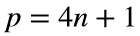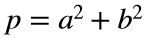# Problem 45964. Compute the nth Pythagorean prime

Pythagorean primes have the form, where n is an integer, and they can be written as the sum of squares of two integers. More information is available at Wikipedia, Numberphile, and the Online Encyclopedia of Integer Sequences.
Compute the nth Pythagorean prime p and two integers a and b such that.

### Solution Stats

43.75% Correct | 56.25% Incorrect
Last Solution submitted on Aug 17, 2023

### Community Treasure Hunt

Find the treasures in MATLAB Central and discover how the community can help you!

Start Hunting!